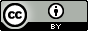# Seward, J.R.; Cronin, M.T.D.; Schultz, T.W. Structure-Toxicity Analyses of Tetrahymena Pyriformis Exposed to Pyridines - An Examination Into Extension of Surface-Response Domains. SAR QSAR Environ. Res. 2001, 11, 5, 489–512.

## QsarDB content

### Property pIGC50: 40-h Tetrahymena toxicity as log(1/IGC50) [log(L/mmol)]

##### 3: All pyridines

Regression model (regression)

Open in:QDB Explorer QDB Predictor

Name Type n

R2

σ

Training training 99 0.610 0.528
##### 4: Selected pyridines

Regression model (regression)

Open in:QDB Explorer QDB Predictor

Name Type n

R2

σ

Training training 83 0.756 0.370
Validation external validation 10 0.662 0.507
##### 5: All pyridines

Regression model (regression)

Open in:QDB Explorer QDB Predictor

Name Type n

R2

σ

Training training 99 0.585 0.545
##### 6: Selected pyridines

Regression model (regression)

Open in:QDB Explorer QDB Predictor

Name Type n

R2

σ

Training training 87 0.734 0.389
Validation external validation 10 0.708 0.458

## Citing

When using this QDB archive, please cite (see details) it together with the original article:

• Ruusmann, V. Data for: Structure-Toxicity Analyses of Tetrahymena Pyriformis Exposed to Pyridines - An Examination Into Extension of Surface-Response Domains. QsarDB repository, QDB.72. 2012. http://dx.doi.org/10.15152/QDB.72

• Seward, J. R.; Cronin, M. T. D.; Schultz, T. W. Structure-Toxicity Analyses of Tetrahymena Pyriformis Exposed to Pyridines - An Examination Into Extension of Surface-Response Domains. SAR QSAR Environ. Res. 2001, 11, 489–512. http://dx.doi.org/10.1080/10629360108035365

 Title: Seward, J.R.; Cronin, M.T.D.; Schultz, T.W. Structure-Toxicity Analyses of Tetrahymena Pyriformis Exposed to Pyridines - An Examination Into Extension of Surface-Response Domains. SAR QSAR Environ. Res. 2001, 11, 5, 489–512. Abstract: A selection of mechanistically diverse substituted pyridines were tested in the Tetrahymena pyriformis population growth impairment assay. The response-surface approach was used to derive multiple-regression type structure-toxicity relationships between T. pyriformis population growth impairment toxicity data (log(IGC(-1)(50)) and the 1-octanol/water partition coefficient (log K(ow)) and one of two different descriptors of molecular orbital interaction: energy of the lowest unoccupied molecular orbital (E(LUMO)) and maximum acceptor superdelocalizability (S(MAX)). A statistically robust model (log(IGC(-1)(50)) = -3.91 + 0.50(logK(ow)) + 10.70(S(MAX)); n=83, r(2) =0.756, s=0.38, F=124, Pr>F=0.0001) was developed with S(MAX) as the indicator of reactivity. This model was not statistically different in fit from the model (log(IGC(-1)(50)) = -1.19 = 0.56(logK(ow)) - 0.61(E(LUMO)); n=86, r(2) =0.749, s=0.38, F=124, Pr>F=0.0001) derived using the alternative descriptor of electrophilic interaction. Compounds with high residual values were removed. An examination of these outliers from both response-surfaces, revealed that pyridines substituted in the 2-position with electron-releasing groups and halogenated nitro-substituted pyridines did not fit the above models well. A third group of outliers, the mono-halogenated pyridines, was unique to the S(MAX) response-surface, which are neutral narcotics with potentially high volatility. A comparison of observed and predicted toxicities for a validation set of pyridines for the S(MAX) surface (log(observed IGC(-1)(50) = 0.10 + 0.75(log(predicted IGC(-1)(50)); n=10, r(2) =0.662, s=0.49, F=15.7, Pr>F=0.004) and the E(LUMO) surface (log(observed IGC(-1)(50)) = 0.17 + 0.80(log(predicted IGC(-1)(50)); n=10, r(2) =0.707, s=0.45, F=19.3, Pr>F=0.002) validated the above models, with the fit in the same range as the parent model. The model derived with S(MAX) was compared to the response-surface derived for substituted benzenes (log(IGC(-1)(50)) = -3.47 + 0.50(logK(ow)) + 9.85(S(MAX)); n=197, r(2) =0.816, s=0.34, F=429, Pr>F=0.0001) revealing the similarities in slope and intercept between the two response-surfaces. The model fit was poorer for the pyridine surface, which may be a factor of increased reactivity due to the presence of nitrogen and the associated pair of unshared electrons in the ring not present in benzene. However, the similarity of the pyridine and benzene response-surfaces suggests that the domain defined for benzenes may be extended to encompass nitrogen heterocyclic pyridines. URI: http://hdl.handle.net/10967/72 http://dx.doi.org/10.15152/QDB.72 Date: 2012-05-23
﻿

## Files in this item

Name Description Format Size View
757084385.qdb.zip n/a application/zip 12.58Kb View/OpenFiles associated with this item are distributed